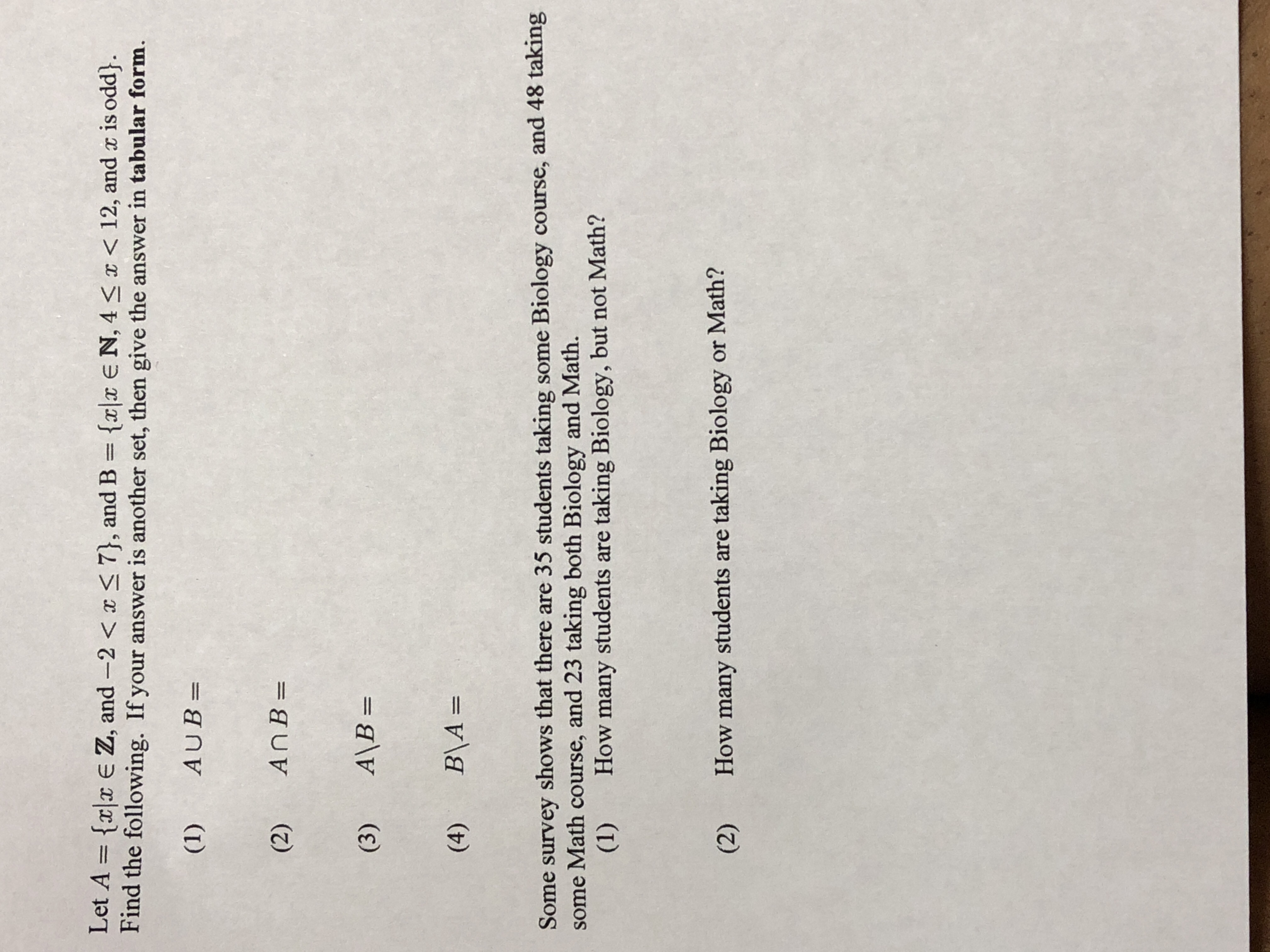# Let A = {xlx E Z, and-2 < x 7), and B = {x|x E N, 4 x < 12, and x is odd) Find the following. If your answer is another set, then give the answer in tabular form (1) AUB- (2) An B- (3) AB- (4) B14 Some survey shows that there are 35 students taking some Biology course, and 48 taking some Math course, and 23 taking both Biology and Math. (1) How many students are taking Biology, but not Math? (2) How many students are taking Biology or Math?

Questionhelp_outlineImage TranscriptioncloseLet A = {xlx E Z, and-2 < x 7), and B = {x|x E N, 4 x < 12, and x is odd) Find the following. If your answer is another set, then give the answer in tabular form (1) AUB- (2) An B- (3) AB- (4) B14 Some survey shows that there are 35 students taking some Biology course, and 48 taking some Math course, and 23 taking both Biology and Math. (1) How many students are taking Biology, but not Math? (2) How many students are taking Biology or Math? fullscreen